In :
import numpy as np
import matplotlib.pyplot as plt
%matplotlib inline
from IPython.display import Image
import pandas as pd

Lecture 18:¶

• start to make some basic maps using Cartopy. Yippee (we love maps).

Introduction to maps¶

Maps in Python used to be plotted using the tools in matplotlib's Basemap module. But Basemap is being deprecated, so we are switching to a new, actively developed toolkit called cartopy.

The first thing we have to do is import a bunch of stuff from cartopy and from matplotlib (assuming you have cartopy installed - if not, install at least cartopy=0.17.0) by typing this on your command line:

conda install cartopy=0.17.0

Here's what we need to make maps with cartopy:

In :
import cartopy
import cartopy.crs as ccrs
from cartopy import config
from cartopy.mpl.ticker import LongitudeFormatter, LatitudeFormatter
from cartopy.mpl.gridliner import LONGITUDE_FORMATTER, LATITUDE_FORMATTER
from cartopy import feature as cfeature
from cartopy.feature import NaturalEarthFeature, LAND, COASTLINE, OCEAN, LAKES, BORDERS
import matplotlib.ticker as mticker

There are many different types of maps used in the Earth Sciences. A map tries to represent something that is essentially 3D (a globe) onto a 2D medium (paper or a computer screen). So all maps, except those at the smallest scale, will increasingly distort the area as the scale increases because the Earth is not 2-dimensional. [No the Earth is not flat! https://en.wikipedia.org/wiki/Modern_flat_Earth_societies]

When we choose a map projection, we seek the one that distorts the least for our purpose.

Here we will use a few popular projections to make maps on both the global and local scale. Let's start with the global projections.

Mercator Projection¶

The one probably everyone is familiar with is the standard Mercator Projection:

In :
Image(filename='Figures/mercator.jpg',width=500)
Out: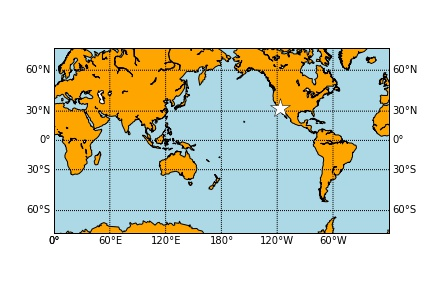In cartopy maps are instances of the Axes figure class. They have many methods, for example outlining continents, national boundaries, and state boundaries and plotting geospatial data, such as sampling locations, earthquakes, and much else.

Before we plot can plot a set of coordinates, they must be transformed from latitudes and longitudes to map coordinates and then plotted like anything else in matplotlib.

To make a map instance, we use the projection keyword in the plt.axes( ) object (normally ax).

To set up the map, you need to know what type of projection you want (there are a growing number to choose from) and depending on the projection type, you need to know the map boundaries and other particulars.

We are starting with the Mercator projection. To do this, we set the projection keyword to 'ccrs.Mercator'.

In :
help(ccrs.Mercator)
Help on class Mercator in module cartopy.crs:

class Mercator(Projection)
|  A Mercator projection.
|
|  Method resolution order:
|      Mercator
|      Projection
|      cartopy._crs.CRS
|      builtins.object
|
|  Methods defined here:
|
|  __eq__(self, other)
|      Return self==value.
|
|  __hash__(self)
|      Hash the CRS based on its proj4_init string.
|
|  __init__(self, central_longitude=0.0, min_latitude=-80.0, max_latitude=84.0, globe=None, latitude_true_scale=None, false_easting=0.0, false_northing=0.0, scale_factor=None)
|      Parameters
|      ----------
|      central_longitude: optional
|          The central longitude. Defaults to 0.
|      min_latitude: optional
|          The maximum southerly extent of the projection. Defaults
|          to -80 degrees.
|      max_latitude: optional
|          The maximum northerly extent of the projection. Defaults
|          to 84 degrees.
|      globe: A :class:cartopy.crs.Globe, optional
|          If omitted, a default globe is created.
|      latitude_true_scale: optional
|          The latitude where the scale is 1. Defaults to 0 degrees.
|      false_easting: optional
|          X offset from the planar origin in metres. Defaults to 0.
|      false_northing: optional
|          Y offset from the planar origin in metres. Defaults to 0.
|      scale_factor: optional
|          Scale factor at natural origin. Defaults to unused.
|
|      Notes
|      -----
|      Only one of latitude_true_scale and scale_factor should
|      be included.
|
|  __ne__(self, other)
|      Return self!=value.
|
|  ----------------------------------------------------------------------
|  Data descriptors defined here:
|
|  boundary
|
|  threshold
|
|  x_limits
|
|  y_limits
|
|  ----------------------------------------------------------------------
|  Data and other attributes defined here:
|
|
|  __abstractmethods__ = frozenset()
|
|  ----------------------------------------------------------------------
|  Methods inherited from Projection:
|
|  project_geometry(self, geometry, src_crs=None)
|      Project the given geometry into this projection.
|
|      Parameters
|      ----------
|      geometry
|          The geometry to (re-)project.
|      src_crs: optional
|          The source CRS.  Defaults to None.
|
|          If src_crs is None, the source CRS is assumed to be a geodetic
|          version of the target CRS.
|
|      Returns
|      -------
|      geometry
|          The projected result (a shapely geometry).
|
|  quick_vertices_transform(self, vertices, src_crs)
|      Where possible, return a vertices array transformed to this CRS from
|      the given vertices array of shape (n, 2) and the source CRS.
|
|      Note
|      ----
|          This method may return None to indicate that the vertices cannot
|          be transformed quickly, and a more complex geometry transformation
|          is required (see :meth:cartopy.crs.Projection.project_geometry).
|
|  ----------------------------------------------------------------------
|  Data descriptors inherited from Projection:
|
|  __dict__
|      dictionary for instance variables (if defined)
|
|  __weakref__
|      list of weak references to the object (if defined)
|
|  ccw_boundary
|
|  cw_boundary
|
|  domain
|
|  ----------------------------------------------------------------------
|  Methods inherited from cartopy._crs.CRS:
|
|  __ge__(self, value, /)
|      Return self>=value.
|
|  __getstate__(...)
|      CRS.__getstate__(self)
|      Return the full state of this instance for reconstruction
|              in __setstate__.
|
|  __gt__(self, value, /)
|      Return self>value.
|
|  __le__(self, value, /)
|      Return self<=value.
|
|  __lt__(self, value, /)
|      Return self<value.
|
|  __new__(*args, **kwargs) from builtins.type
|      Create and return a new object.  See help(type) for accurate signature.
|
|  __reduce__(...)
|      CRS.__reduce__(self)
|
|      Implement the __reduce__ API so that unpickling produces a stateless
|      instance of this class (e.g. an empty tuple). The state will then be
|      added via __getstate__ and __setstate__.
|
|  __setstate__(...)
|      CRS.__setstate__(self, state)
|
|      Take the dictionary created by __getstate__ and passes it
|      through to the class's __init__ method.
|
|  as_geocentric(...)
|      CRS.as_geocentric(self)
|
|      Return a new Geocentric CRS with the same ellipse/datum as this
|      CRS.
|
|  as_geodetic(...)
|      CRS.as_geodetic(self)
|
|      Return a new Geodetic CRS with the same ellipse/datum as this
|      CRS.
|
|  is_geodetic(...)
|      CRS.is_geodetic(self)
|
|  transform_point(...)
|      CRS.transform_point(self, double x, double y, CRS src_crs, trap=True)
|
|      transform_point(x, y, src_crs)
|
|      Transform the given float64 coordinate pair, in the given source
|      coordinate system (src_crs), to this coordinate system.
|
|      Parameters
|      ----------
|      x
|          the x coordinate, in src_crs coordinates, to transform
|      y
|          the y coordinate, in src_crs coordinates, to transform
|      src_crs
|          instance of :class:CRS that represents the coordinate
|          system of x and y.
|      trap
|          Whether proj errors for "latitude or longitude exceeded limits" and
|          "tolerance condition error" should be trapped.
|
|      Returns
|      -------
|      (x, y) in this coordinate system
|
|  transform_points(...)
|      CRS.transform_points(self, CRS src_crs, ndarray x, ndarray y, ndarray z=None)
|
|      transform_points(src_crs, x, y[, z])
|
|      Transform the given coordinates, in the given source
|      coordinate system (src_crs), to this coordinate system.
|
|      Parameters
|      ----------
|      src_crs
|          instance of :class:CRS that represents the
|          coordinate system of x, y and z.
|      x
|          the x coordinates (array), in src_crs coordinates,
|          to transform.  May be 1 or 2 dimensional.
|      y
|          the y coordinates (array), in src_crs coordinates,
|          to transform.  Its shape must match that of x.
|      z: optional
|          the z coordinates (array), in src_crs coordinates, to
|          transform.  Defaults to None.
|          If supplied, its shape must match that of x.
|
|      Returns
|      -------
|          Array of shape x.shape + (3, ) in this coordinate system.
|
|  transform_vectors(...)
|      CRS.transform_vectors(self, src_proj, x, y, u, v)
|
|      transform_vectors(src_proj, x, y, u, v)
|
|      Transform the given vector components, with coordinates in the
|      given source coordinate system (src_proj), to this coordinate
|      system. The vector components must be given relative to the
|      source projection's coordinate reference system (grid eastward and
|      grid northward).
|
|      Parameters
|      ----------
|      src_proj
|          The :class:CRS.Projection that represents the coordinate system
|          the vectors are defined in.
|      x
|          The x coordinates of the vectors in the source projection.
|      y
|          The y coordinates of the vectors in the source projection.
|      u
|          The grid-eastward components of the vectors.
|      v
|          The grid-northward components of the vectors.
|
|      Note
|      ----
|          x, y, u and v may be 1 or 2 dimensional, but must all have matching
|          shapes.
|
|      Returns
|      -------
|          ut, vt: The transformed vector components.
|
|      Note
|      ----
|         The algorithm used to transform vectors is an approximation
|         rather than an exact transform, but the accuracy should be
|         good enough for visualization purposes.
|
|  ----------------------------------------------------------------------
|  Data descriptors inherited from cartopy._crs.CRS:
|
|  proj4_init
|
|  proj4_params
|
|  ----------------------------------------------------------------------
|  Data and other attributes inherited from cartopy._crs.CRS:
|
|  __pyx_vtable__ = <capsule object NULL>

So we need: central_longitude, min_latitude, and max_latitude

We make the map instance with a call like:

ax = plt.axes(projection=ccrs.Mercator(central_longitude=180.0, min_latitude=-70.0, max_latitude=70.0, globe=None))

We also have to put something on the map, so let's draw the coastlines by using the coastlines() method:

ax.coastlines()

Here's our basic Mercator map of the whole world:

In :
ax = plt.axes(projection=ccrs.Mercator(
central_longitude=180.0, min_latitude=-70.0, max_latitude=70.0, globe=None))
ax.coastlines();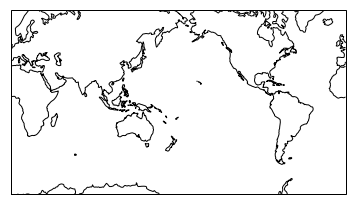We usually want lines of latitude (parallels) and longitude (meridians) on there as well, so we use the gridlines( ) method with the keyword crs=ccrs.PlateCarree( ) to make a globe object (usually called $g$ or $g1$.

In :
help(ax.gridlines)
Help on method gridlines in module cartopy.mpl.geoaxes:

gridlines(crs=None, draw_labels=False, xlocs=None, ylocs=None, **kwargs) method of cartopy.mpl.geoaxes.GeoAxesSubplot instance
Automatically add gridlines to the axes, in the given coordinate
system, at draw time.

Parameters
----------
crs: optional
The :class:cartopy._crs.CRS defining the coordinate system in
which gridlines are drawn.
Defaults to :class:cartopy.crs.PlateCarree.
draw_labels: optional
Label gridlines like axis ticks, around the edge.
xlocs: optional
An iterable of gridline locations or a
:class:matplotlib.ticker.Locator instance which will be
used to determine the locations of the gridlines in the
x-coordinate of the given CRS. Defaults to None, which
implies automatic locating of the gridlines.
ylocs: optional
An iterable of gridline locations or a
:class:matplotlib.ticker.Locator instance which will be
used to determine the locations of the gridlines in the
y-coordinate of the given CRS. Defaults to None, which
implies automatic locating of the gridlines.

Returns
-------
gridliner
A :class:cartopy.mpl.gridliner.Gridliner instance.

Note
----
All other keywords control line properties.  These are passed
through to :class:matplotlib.collections.Collection.

Now we stick the latitudes and longitudes onto our globe object ($g1$ in the following example). We will need the matplotlib.ticker module (already imported as mticker) which has the useful attributes mticker.FixedLocator( ) method which allows us to make an create a special object of latitudes or longitudes with the desired spacing (using our old friend np.arange( )). These can be attached to the figure object using figure attributes g1.ylocator and g1.xlocator for latitudes and longitudes respectively. (You'll see how this works soon, so be a little patient!).

The steps are:

1) Make the figure object ($ax$) with the desired projection using plt.axes( ).

2) Put on the coastlines

3) Make the globe object ($g1$) using ax.gridlines( )

4) Put on the latitude and longitudes by setting the g1.ylocator and g1.xlocator attributes to the desired mticker.FixedLocator(ARRAY) object.

Here we go:

In :
# make the figure object
ax = plt.axes(projection=ccrs.Mercator(
central_longitude=180.0, min_latitude=-70.0, max_latitude=70.0, globe=None))
# put on the coastlines
ax.coastlines()
# make the globe object
gl=ax.gridlines(crs=ccrs.PlateCarree(),linewidth=2,linestyle='dotted')
# put on the latitudes
gl.ylocator=mticker.FixedLocator(np.arange(-90,91,30))
# put on the longitudes
gl.xlocator=mticker.FixedLocator(np.arange(-180.,181.,60.));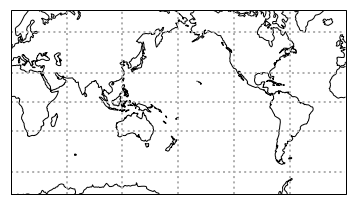And surely you want the lat/long labels to show, so you can modify your commands like this:

In :
ax = plt.axes(projection=ccrs.Mercator(
central_longitude=180.0, min_latitude=-70.0, max_latitude=70.0, globe=None))
ax.coastlines()
g1=ax.gridlines(crs=ccrs.PlateCarree(),linewidth=2,linestyle='dotted',draw_labels=True)
g1.ylocator=mticker.FixedLocator(np.arange(-90,91,30))
g1.xlocator=mticker.FixedLocator(np.arange(-180.,181,60.))
g1.xformatter = LONGITUDE_FORMATTER
g1.yformatter = LATITUDE_FORMATTER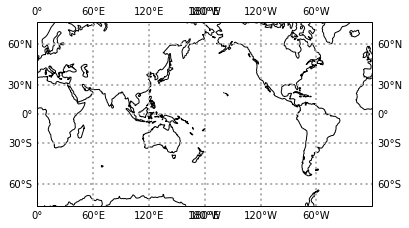It seems that you can either have a gap in the latitude lines (as in this example), or a doubled 180$^{\circ}$W/180$^{\circ}$E. This is a "feature". But I did find a workaround for this special case. You draw the gridlines separately from the labels. We don't get the nice degree labels, but at least we don't get the doubled 180 tick mark!

In :
ax = plt.axes(projection=ccrs.Mercator(
central_longitude=180.0, min_latitude=-70.0, max_latitude=70.0, globe=None))
ax.coastlines()
ylabels=np.arange(-90,91,30)
xlabels=np.arange(-180.,181,60.)
g1=ax.gridlines(xlocs=xlabels,ylocs=ylabels, crs=ccrs.PlateCarree(),linewidth=2,linestyle='dotted',draw_labels=False)
xlabels=np.arange(-180.,180,60.)
glabels = ax.gridlines(xlocs=xlabels,
ylocs=ylabels,
draw_labels=True, alpha=0)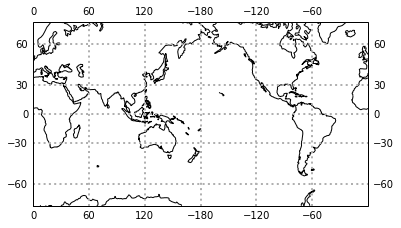To turn labels off for the top, bottom, left or right sides, we can use attributes of the globe object like this:

In :
ax = plt.axes(projection=ccrs.Mercator(
central_longitude=180.0, min_latitude=-70.0, max_latitude=70.0, globe=None))
ax.coastlines()
ylabels=np.arange(-90,91,30)
xlabels=np.arange(-180.,181,60.)
g1=ax.gridlines(xlocs=xlabels,ylocs=ylabels, crs=ccrs.PlateCarree(),linewidth=2,linestyle='dotted',draw_labels=False)
xlabels=np.arange(-180.,180,60.)
glabels = ax.gridlines(xlocs=xlabels,
ylocs=ylabels,
draw_labels=True, alpha=0)
glabels.xlabels_top = False
glabels.ylabels_right = False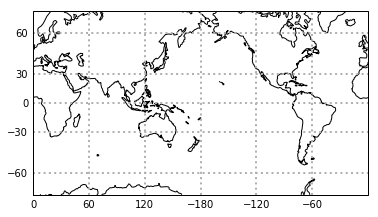Now maybe you want some color? You can set the color of the continents with:

and color in the oceans with:

In :
ax = plt.axes(projection=ccrs.Mercator(
central_longitude=180.0, min_latitude=-70.0, max_latitude=70.0, globe=None))
ylabels=np.arange(-90,91,30)
xlabels=np.arange(-180.,181,60.)
g1=ax.gridlines(xlocs=xlabels,ylocs=ylabels, crs=ccrs.PlateCarree(),
linewidth=2,linestyle='dotted',draw_labels=False)
xlabels=np.arange(-180.,180,60.)
glabels = ax.gridlines(xlocs=xlabels,
ylocs=ylabels,
draw_labels=True, alpha=0)
glabels.xlabels_top = False
ax.coastlines(); # this has to come last, or it is buried by the ocean, land colors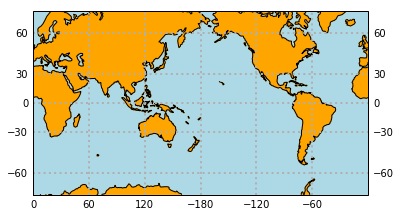The lakes are missing! You can change lake colors using the LAKES attribute if you so desire. You can set the facecolor and edgecolor separately.

You also want to put something ON the map? No problem. Just take your latitude and longitude (or arrays of them) and convert them to map coordinates using the transform=ccrs.Geodetic( ) keyword in the plt.plot( ) command. These you plot just like any ordinary matplotlib plot, except you need a ax.set_global( ) command after the plot, or you get a map near San Diego (for some reason...).

Let's plot the position of San Diego as a big white star with the marker="*", markersize=20, and color = 'white' arguments.

In :
ax = plt.axes(projection=ccrs.Mercator(
central_longitude=180.0, min_latitude=-70.0, max_latitude=70.0, globe=None))
ylabels=np.arange(-90,91,30)
xlabels=np.arange(-180.,181,60.)
g1=ax.gridlines(xlocs=xlabels,ylocs=ylabels, crs=ccrs.PlateCarree(),
linewidth=2,linestyle='dotted',draw_labels=False)
xlabels=np.arange(-180.,180,60.)
glabels = ax.gridlines(xlocs=xlabels,
ylocs=ylabels,
draw_labels=True, alpha=0)
glabels.xlabels_top = False
ax.coastlines()

San_lat=33
San_lon=-117%360  # takes the west longitude and converts to 0=>360

ax.plot([San_lon],[San_lat],marker='*',color='white',\
markersize=20,transform=ccrs.Geodetic(),markeredgecolor='black')
ax.set_global() # we need this or weird things happen
ax.coastlines();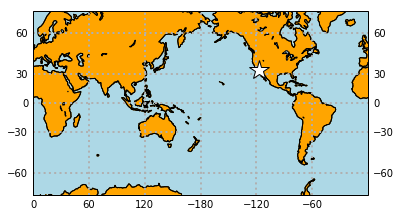Orthographic Projection¶

The Mercator is a nice classical map, but it sure does distort the map at high latitudes. Think back to the lecture on the hypsometric curves...

Another type of map projection is the orthographic projection which is much less distorted. The downside to this projection is that you cannot see the whole globe at once. To create an orthographic map, you initialize a map instance with the projection set to ccrs.Orthographic and a tuple of the central longitude and latitude.

In :
ax = plt.axes(projection=ccrs.Orthographic(-75, 42))
ax.coastlines();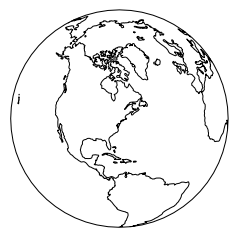And finish the map as before.

In :
ax = plt.axes(projection=ccrs.Orthographic(-75, 42))
San_lat=33
San_lon=-117%360  # takes the west longitude and converts to 0=>360 using modular syntax
gl=ax.gridlines(crs=ccrs.PlateCarree(),color='black',linewidth=1,linestyle='dotted')
#gl.xlabels_top = False
gl.ylocator=mticker.FixedLocator(np.arange(-80,81,20))
gl.xlocator=mticker.FixedLocator(np.arange(-180,181,60));
gl.xformatter = LONGITUDE_FORMATTER
gl.yformatter = LATITUDE_FORMATTER
ax.plot([San_lon],[San_lat],marker='*',color='white',\
markersize=20,transform=ccrs.Geodetic(),markeredgecolor='black')
ax.set_global()
ax.coastlines();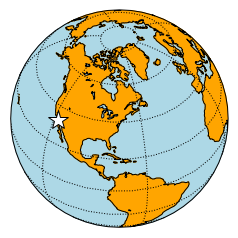Mollweide projection¶

One more global scale example of a map projection is the Hammer projection (one of my favorites). Unfortunately, cartopy does not have a Hammer projection (yet), so we will learn about a Mollweide projection instead. And really it is pretty similar, so no worries. The Mollweide projection is always a global map centered on the equator, so all you need to specify is the central longitude (with the central_longitude keyword). Note that any central_longitude but 180 is super slow for some reason...

In :
#no Hammer in cartopy but yes mollweide
ax = plt.axes(projection=ccrs.Mollweide(central_longitude=180))
San_lat=33
San_lon=-117%360  # takes the west longitude and converts to 0=>360
gl=ax.gridlines(crs=ccrs.PlateCarree(),color='black',linewidth=1,linestyle='dotted')
gl.xlabels_top = False
gl.ylocator=mticker.FixedLocator(np.arange(-90,91,30))
gl.xlocator=mticker.FixedLocator(np.arange(0,400,30));
gl.xformatter = LONGITUDE_FORMATTER
gl.yformatter = LATITUDE_FORMATTER
ax.plot([San_lon],[San_lat],marker='*',color='white',\
markersize=20,transform=ccrs.Geodetic(),markeredgecolor='black')
ax.set_global()

ax.coastlines();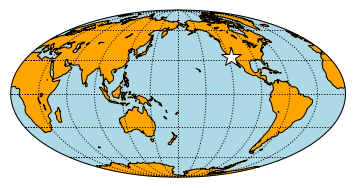Lambert conformal¶

The maps we've explored so far are well and good for global scale problems, for example plotting the locations of earthquakes around the globe, but not so great for more local problems, like a map of sampling sites. For this we need a smaller scale map and the Lambert confomal conic projection is a popular choice. For this we must specify the central latitude and longitude and the map boundaries (with ax.set_extent( ).

Here's what we know so far:

In :
# cannot get labels for this projection yet
proj = ccrs.LambertConformal(central_longitude=260, central_latitude=33)
ax = plt.axes(projection=proj)
ax.set_extent([-130, -70, 20, 52], crs=ccrs.PlateCarree())
ax.plot([San_lon],[San_lat],marker='*',color='white',\
markersize=20,transform=ccrs.Geodetic(),markeredgecolor='black')
gl=ax.gridlines(ylocs=np.arange(0,90,15.),xlocs=np.arange(-180.,180,15.),\
linewidth=2, linestyle="dotted")

ax.coastlines();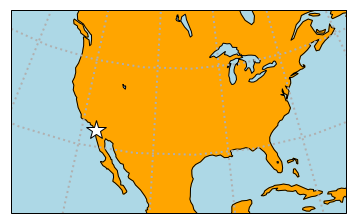One problem is that cartopy does not support tick labels in the Lambert projection. I found this work around online (https://gist.github.com/ajdawson/dd536f786741e987ae4e) and let's see if it works.

In :
import shapely.geometry as sgeom
from copy import copy
def find_side(ls, side):
"""
Given a shapely LineString which is assumed to be rectangular, return the
line corresponding to a given side of the rectangle.

"""
minx, miny, maxx, maxy = ls.bounds
points = {'left': [(minx, miny), (minx, maxy)],
'right': [(maxx, miny), (maxx, maxy)],
'bottom': [(minx, miny), (maxx, miny)],
'top': [(minx, maxy), (maxx, maxy)],}
return sgeom.LineString(points[side])

def lambert_xticks(ax, ticks):
"""Draw ticks on the bottom x-axis of a Lambert Conformal projection."""
te = lambda xy: xy
lc = lambda t, n, b: np.vstack((np.zeros(n) + t, np.linspace(b, b, n))).T
xticks, xticklabels = _lambert_ticks(ax, ticks, 'bottom', lc, te)
ax.xaxis.tick_bottom()
ax.set_xticks(xticks)
ax.set_xticklabels([ax.xaxis.get_major_formatter()(xtick) for xtick in xticklabels])

def lambert_yticks(ax, ticks):
"""Draw ricks on the left y-axis of a Lamber Conformal projection."""
te = lambda xy: xy
lc = lambda t, n, b: np.vstack((np.linspace(b, b, n), np.zeros(n) + t)).T
yticks, yticklabels = _lambert_ticks(ax, ticks, 'left', lc, te)
ax.yaxis.tick_left()
ax.set_yticks(yticks)
ax.set_yticklabels([ax.yaxis.get_major_formatter()(ytick) for ytick in yticklabels])

def _lambert_ticks(ax, ticks, tick_location, line_constructor, tick_extractor):
"""Get the tick locations and labels for an axis of a Lambert Conformal projection."""
outline_patch = sgeom.LineString(ax.outline_patch.get_path().vertices.tolist())
axis = find_side(outline_patch, tick_location)
n_steps = 30
extent = ax.get_extent(ccrs.PlateCarree())
_ticks = []
for t in ticks:
xy = line_constructor(t, n_steps, extent)
proj_xyz = ax.projection.transform_points(ccrs.Geodetic(), xy[:, 0], xy[:, 1])
xyt = proj_xyz[..., :2]
ls = sgeom.LineString(xyt.tolist())
locs = axis.intersection(ls)
if not locs:
tick = [None]
else:
tick = tick_extractor(locs.xy)
_ticks.append(tick)
# Remove ticks that aren't visible:
ticklabels = copy(ticks)
while True:
try:
index = _ticks.index(None)
except ValueError:
break
_ticks.pop(index)
ticklabels.pop(index)
return _ticks, ticklabels
In :
proj = ccrs.LambertConformal(central_longitude=260, central_latitude=33)
fig = plt.figure(figsize=(6,6), frameon=True) # you need this frameon to be true
ax = plt.axes(projection=proj)
ax.set_extent([-130, -70, 20, 52], crs=ccrs.PlateCarree())
ax.plot([San_lon],[San_lat],marker='*',color='white',\
markersize=20,transform=ccrs.Geodetic(),markeredgecolor='black')

# *must* call draw in order to get the axis boundary used to add ticks:
fig.canvas.draw()

xticks=list(range(-180,-30,15))
yticks=list(range(0,90,15))

ax.gridlines(ylocs=yticks,xlocs=xticks,linewidth=2, linestyle="dotted")
ax.xaxis.set_major_formatter(LONGITUDE_FORMATTER) # you need this here
ax.yaxis.set_major_formatter(LATITUDE_FORMATTER)# you need this here, too

lambert_xticks(ax, xticks)
lambert_yticks(ax, yticks)

ax.coastlines();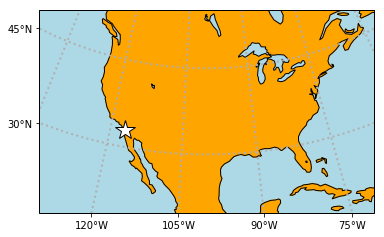Oh my, that is lovely!

But it sure would be nice to put on national and state boundaries. We can draw the national boundaries in a thick line like this:

In :
proj = ccrs.LambertConformal(central_longitude=260, central_latitude=33)
fig = plt.figure(figsize=(6,6), frameon=True) # you need this frameon to be true
ax = plt.axes(projection=proj)
ax.set_extent([-130, -70, 20, 52], crs=ccrs.PlateCarree())
ax.plot([San_lon],[San_lat],marker='*',color='white',\
markersize=20,transform=ccrs.Geodetic(),markeredgecolor='black')

# *must* call draw in order to get the axis boundary used to add ticks:
fig.canvas.draw()

xticks=list(range(-180,-30,15))
yticks=list(range(0,90,15))

ax.gridlines(ylocs=yticks,xlocs=xticks,linewidth=2, linestyle="dotted")
ax.xaxis.set_major_formatter(LONGITUDE_FORMATTER) # you need this here
ax.yaxis.set_major_formatter(LATITUDE_FORMATTER)# you need this here, too

lambert_xticks(ax, xticks)
lambert_yticks(ax, yticks)

ax.coastlines();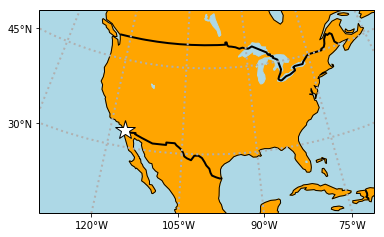And finish off with the state boundaries like this:

In :
proj = ccrs.LambertConformal(central_longitude=260, central_latitude=33)
fig = plt.figure(figsize=(6,6), frameon=True) # you need this frameon to be true
ax = plt.axes(projection=proj)
ax.set_extent([-130, -70, 20, 52], crs=ccrs.PlateCarree())
ax.plot([San_lon],[San_lat],marker='*',color='white',\
markersize=20,transform=ccrs.Geodetic(),markeredgecolor='black')

# *must* call draw in order to get the axis boundary used to add ticks:
fig.canvas.draw()

xticks=list(range(-180,-30,15))
yticks=list(range(0,90,15))

ax.gridlines(ylocs=yticks,xlocs=xticks,linewidth=2, linestyle="dotted")
ax.xaxis.set_major_formatter(LONGITUDE_FORMATTER) # you need this here
ax.yaxis.set_major_formatter(LATITUDE_FORMATTER)# you need this here, too

lambert_xticks(ax, xticks)
lambert_yticks(ax, yticks)

ax.coastlines();
states_provinces = cfeature.NaturalEarthFeature(
category='cultural',
scale='50m',
edgecolor='purple',
facecolor='none',
linestyle='dotted')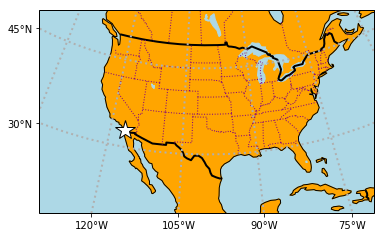You might also want to know that there are other options for background colors but cartopy has a very limited set: the stock image, several Blue Marble version and a shaded relief map:

In :
proj = ccrs.LambertConformal(central_longitude=260, central_latitude=33)
ax = plt.axes(projection=proj)
ax.set_extent([-130, -70, 20, 52], crs=ccrs.PlateCarree())
ax.stock_img();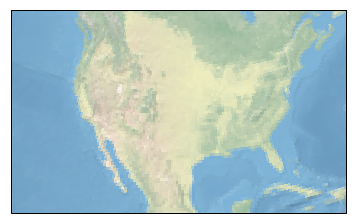For more on background images, see this website: http://earthpy.org/cartopy_backgroung.html

And now for some science!

Earthquake locations - last 5 years¶

Remember the data we've been using with latitude and longitude coordinates? Now we can place those on our maps! Here are all the earthquakes that have occured over a five year period with magnitudes greater than or equal to 5. The data come from: http://earthquake.usgs.gov/earthquakes/search/

Let's take a look:

In :
Out:
['http://earthquake.usgs.gov/fdsnws/event/1/query.csv?starttime=2011-12-09%2000:00:00&endtime=2016-12-16%2023:59:59&minmagnitude=5&orderby=time\n',
'time,latitude,longitude,depth,mag,magType,nst,gap,dmin,rms,net,id,updated,place,type,horizontalError,depthError,magError,magNst,status,locationSource,magSource\n',
'2016-12-15T22:23:28.760Z,-9.9259,160.5101,35,5.3,mb,,32,0.736,1.14,us,us2000815j,2016-12-15T22:40:35.040Z,"82km SE of Honiara, Solomon Islands",earthquake,5.9,2,0.043,184,reviewed,us,us\n',
'2016-12-15T01:29:27.610Z,-29.1659,61.0004,13.71,5.3,mb,,62,9.275,1.29,us,us200080w8,2016-12-15T01:57:38.040Z,"Southwest Indian Ridge",earthquake,10.9,3.6,0.065,80,reviewed,us,us\n',
'2016-12-15T01:06:28.010Z,10.1317,126.1709,80.09,5.1,mb,,103,3.098,1.16,us,us200080vz,2016-12-15T07:52:00.028Z,"16km NE of San Isidro, Philippines",earthquake,10.1,11.1,0.158,13,reviewed,us,us\n']

So we have to skip the first row (by setting the keyword skiprows to 1).

In :
eq_data.columns
Out:
Index(['time', 'latitude', 'longitude', 'depth', 'mag', 'magType', 'nst',
'gap', 'dmin', 'rms', 'net', 'id', 'updated', 'place', 'type',
'horizontalError', 'depthError', 'magError', 'magNst', 'status',
'locationSource', 'magSource'],
dtype='object')

There is a lot of information in this file, but the most important (for now) is the latitude and longitude. We can deal with the depth and magnitude later.

For now, let's extract that location information into arrays.

Remember, this is how you get stuff out of a Pandas DataFrame into NumPy arrays:

In :
lats=eq_data.latitude.values #make an array of the values of a pandas Series
lons=eq_data.longitude.values
lons=lons
print (lats)
print (lons)
[ -9.9259 -29.1659  10.1317 ...  11.077   -0.863   47.009 ]
[160.5101  61.0004 126.1709 ... 125.999  126.907  144.551 ]

Now we can plot these on a Mollweide projection. Everything is just as we did before, except I'm putting on the earthquake locations as white dots. The size of the dots can be set with the keyword markersize.

In :
plt.figure(1,(8,8)) # make a big figure (8x8)
ax = plt.axes(projection=ccrs.Mollweide(central_longitude=180))
gl=ax.gridlines(crs=ccrs.PlateCarree(),color='black',linewidth=1,linestyle='dotted')
gl.xlabels_top = False
gl.ylocator=mticker.FixedLocator(np.arange(-90,91,30))
gl.xlocator=mticker.FixedLocator(np.arange(0,400,30));
gl.xformatter = LONGITUDE_FORMATTER
gl.yformatter = LATITUDE_FORMATTER